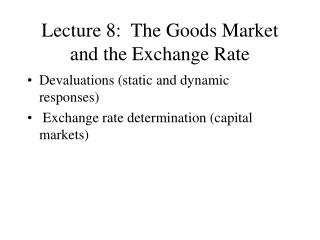# Lecture 8: The Goods Market and the Exchange Rate - PowerPoint PPT PresentationDownload PresentationLecture 8: The Goods Market and the Exchange Rate

Lecture 8: The Goods Market and the Exchange RateDownload Presentation## Lecture 8: The Goods Market and the Exchange Rate

- - - - - - - - - - - - - - - - - - - - - - - - - - - E N D - - - - - - - - - - - - - - - - - - - - - - - - - - -
##### Presentation Transcript

1. Lecture 8: The Goods Market and the Exchange Rate • Devaluations (static and dynamic responses) • Exchange rate determination (capital markets)

2. The Goods Market Z = C + I + G + X - e Q C(Y-T) + I(Y,I) + G Q = Q(Y,e) + - X = X(Y*,e) + +

3. Figures • Figs 19.1 and 19-2 • Increase in domestic and foreign demand • games countries play • depreciation

4. The J-Curve • eQ(Y,e) : increase or decrease with e? • In the very short run: it may increase! • And if strong enough: X(Y*,e) - eQ(Y,e) may do the same. • Dynamics of NX in response to a depreciation; fig 19-6

5. The Exchange Rate The Goods Market Y = C(Y-T) + I(Y,i) + G + NX(Y,Y*, E P*/P) constant Financial Markets M/P = YL(i) i(t) = i*(t) + E(t+1) - E(t) E(t) e

6. Cont. The Exchange Rate i i* e E E e i = i* + E - E E given E and i* e